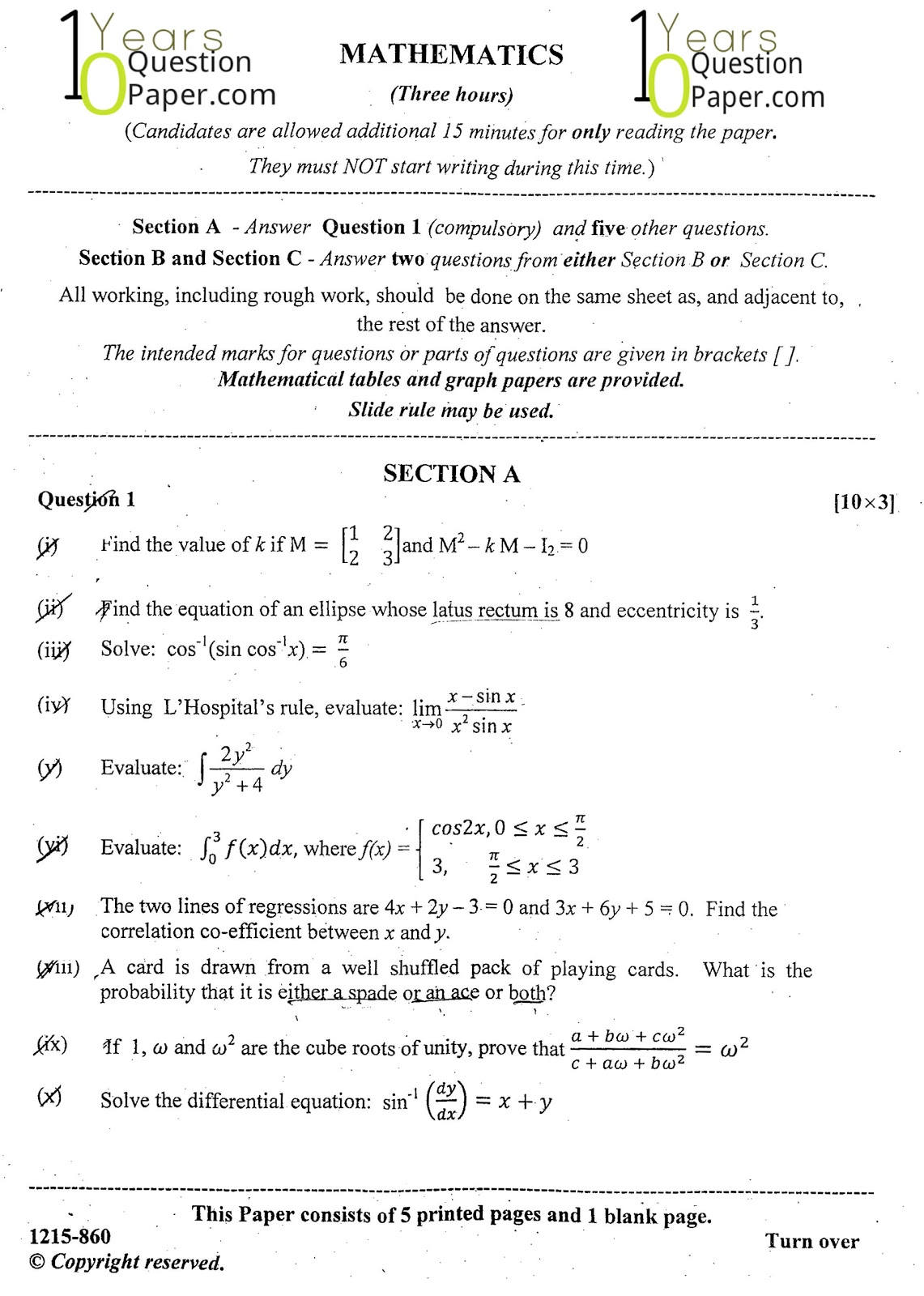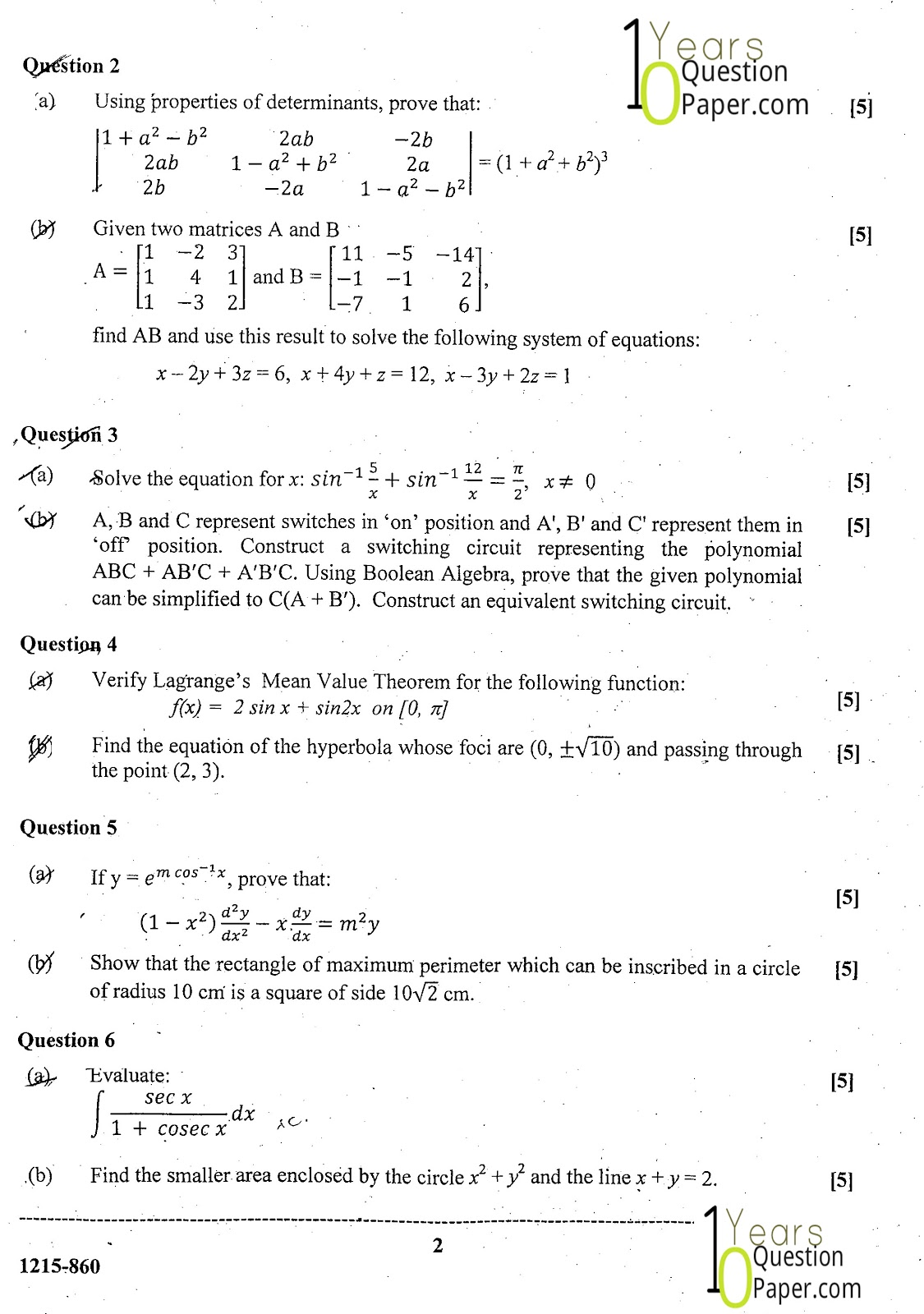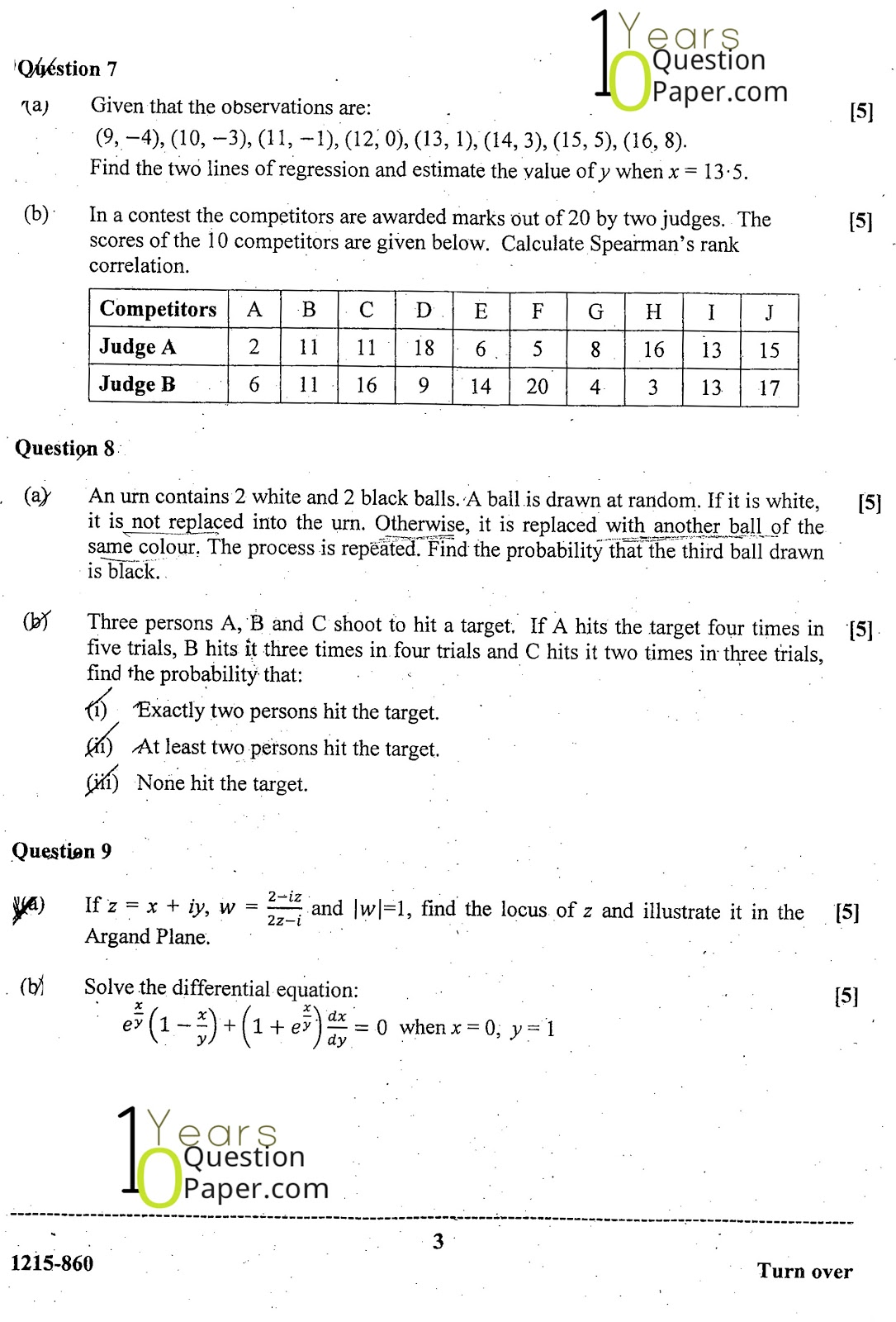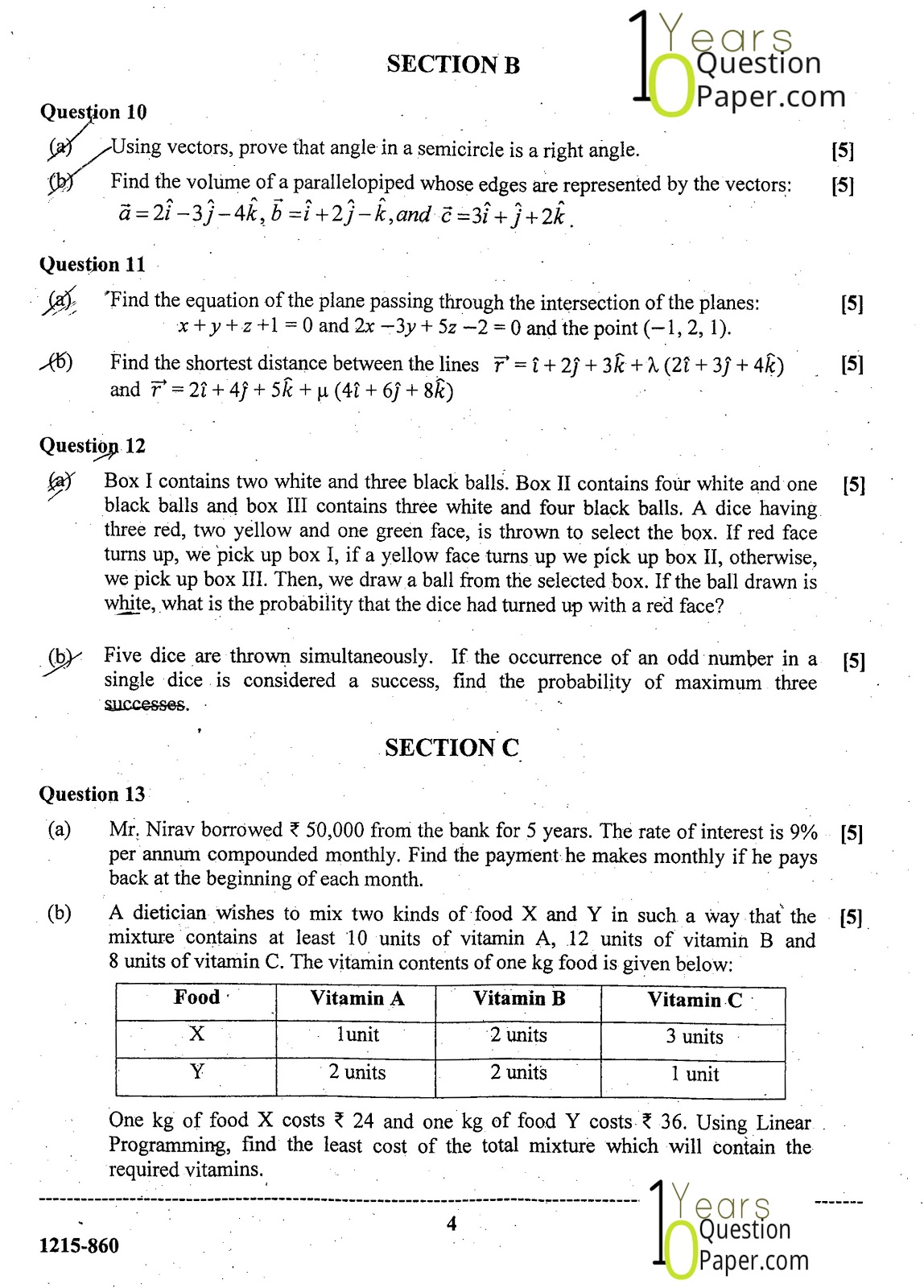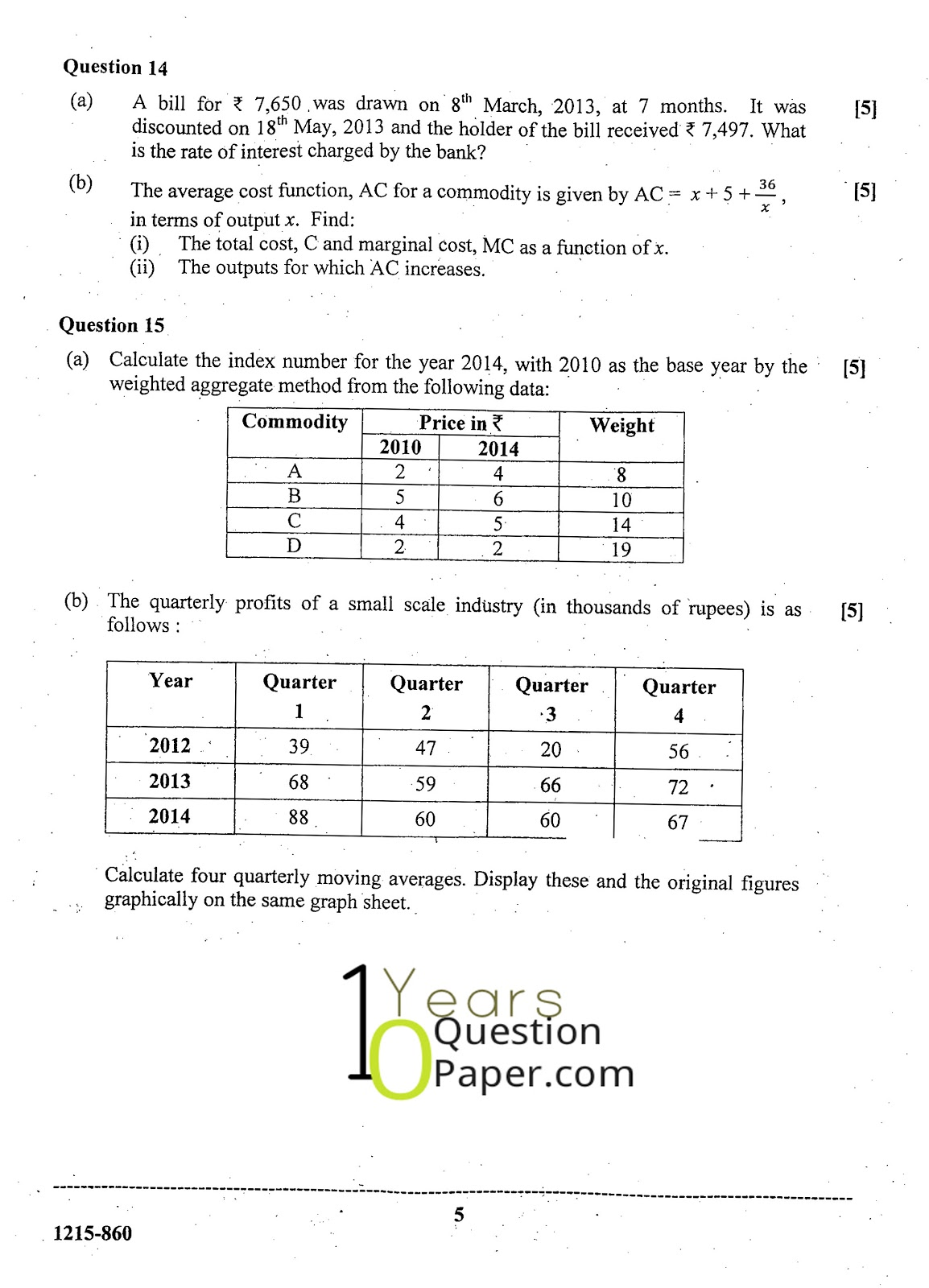# ISC 2015 Mathematics Question Paper for Class 12

Board : ISC    Year : 2015    Subject : Mathematics    Class : 12

ISC 2015 Board Mathematics Paper Class12th free sample Question papers of math 2015 are issued by Indian Certificate Of Secondary Education (i.s.c.). Mathematics of ISC syllabus for class 12 - Determinants and Matrices, Boolean Algebra, Conics, Inverse Trigonometric Function, Calculus, Correlation and Regression, Complex Numbers,Differential Equations, Probability, Vectors, Co-ordinate geometry in 3-Dimensions, Discount,Annuities , Linear Programming, Index numbers and moving averages ,ISC important question and ISC question bank.